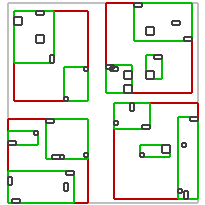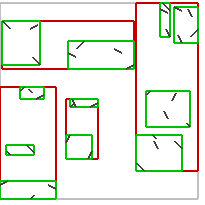#Boost C++ Libraries

...one of the most highly regarded and expertly designed C++ library projects in the world.

### Creation and Modification

##### Template parameters

R-tree has 5 parameters but only 2 are required:

```rtree<Value,
Parameters,
IndexableGetter = index::indexable<Value>,
EqualTo = index::equal_to<Value>,
Allocator = std::allocator<Value> >
```
• `Value` - type of object which will be stored in the container,
• `Parameters` - parameters type, inserting/splitting algorithm,
• `IndexableGetter` - function object translating `Value` to `Indexable` (`Point` or `Box`) which R-tree can handle,
• `EqualTo` - function object comparing `Value`s,
• `Allocator` - `Value`s allocator, all allocators needed by the container are created from it.
##### Values and Indexables

R-tree may store `Value`s of any type as long as passed function objects know how to interpret those `Value`s, that is extract an `Indexable` that the R-tree can handle and compare `Value`s. The `Indexable` is a type adapted to Point, Box or Segment concept. The examples of rtrees storing `Value`s translatable to various `Indexable`s are presented below.

rtree<Point, ...>

rtree<Box, ...>

rtree<Segment, ...>By default function objects `index::indexable<Value>` and `index::equal_to<Value>` are defined for some typically used `Value` types which may be stored without defining any additional classes. By default the rtree may store pure `Indexable`s, pairs and tuples. In the case of those two collection types, the `Indexable` must be the first stored type.

• ```Indexable = Point | Box | Segment```
• ```Value = Indexable | std::pair<Indexable, T> | boost::tuple<Indexable, ...> [ | std::tuple<Indexable, ...> ]```

By default `boost::tuple<...>` is supported on all compilers. If the compiler supports C++11 tuples and variadic templates then `std::tuple<...>` may be used "out of the box" as well.

Examples of default `Value` types:

```geometry::model::point<...>
geometry::model::point_xy<...>
geometry::model::box<...>
geometry::model::segment<...>
std::pair<geometry::model::box<...>, unsigned>
boost::tuple<geometry::model::point<...>, int, float>
```

The predefined `index::indexable<Value>` returns const reference to the `Indexable` stored in the `Value`.

ImportantThe translation is done quite frequently inside the container - each time the rtree needs it.

The predefined `index::equal_to<Value>`:

• for `Point`, `Box` and `Segment` - compares `Value`s with geometry::equals().
• for `std::pair<...>` - compares both components of the `Value`. The first value stored in the pair is compared before the second one. If the value stored in the pair is a Geometry, `geometry::equals()` is used. For other types it uses `operator==()`.
• for `tuple<...>` - compares all components of the `Value`. If the component is a `Geometry`, `geometry::equals()` function is used. For other types it uses `operator==()`.
##### Balancing algorithms compile-time parameters

`Value`s may be inserted to the R-tree in many various ways. Final internal structure of the R-tree depends on algorithms used in the insertion process and parameters. The most important is nodes' balancing algorithm. Currently, three well-known types of R-trees may be created.

Linear - classic R-tree using balancing algorithm of linear complexity

```index::rtree< Value, index::linear<16> > rt;
```

```index::rtree< Value, index::quadratic<16> > rt;
```

R*-tree - balancing algorithm minimizing nodes' overlap with forced reinsertions

```index::rtree< Value, index::rstar<16> > rt;
```
##### Balancing algorithms run-time parameters

Balancing algorithm parameters may be passed to the R-tree in run-time. To use run-time versions of the R-tree one may pass parameters which names start with `dynamic_`.

```// linear
index::rtree<Value, index::dynamic_linear> rt(index::dynamic_linear(16));

// rstar
index::rtree<Value, index::dynamic_rstar> rt(index::dynamic_rstar(16));
```

The obvious drawback is a slightly slower R-tree.

##### Non-default parameters

Non-default R-tree parameters are described in the reference.

##### Copying, moving and swapping

The R-tree is copyable and movable container. Move semantics is implemented using Boost.Move library so it's possible to move the container on a compilers without rvalue references support.

```// default constructor
index::rtree< Value, index::rstar<8> > rt1;

// copy constructor
index::rtree< Value, index::rstar<8> > rt2(r1);

// copy assignment
rt2 = r1;

// move constructor
index::rtree< Value, index::rstar<8> > rt3(boost::move(rt1));

// move assignment
rt3 = boost::move(rt2);

// swap
rt3.swap(rt2);
```
##### Inserting and removing Values

The following code creates an R-tree using quadratic balancing algorithm.

```using namespace boost::geometry;
typedef std::pair<Box, int> Value;
```

To insert or remove a `Value' by method call one may use the following code.

```Value v = std::make_pair(Box(...), 0);

rt.insert(v);

rt.remove(v);
```

To insert or remove a `Value' by function call one may use the following code.

```Value v = std::make_pair(Box(...), 0);

index::insert(rt, v);

index::remove(rt, v);
```

Typically you will perform those operations in a loop in order to e.g. insert some number of `Value`s corresponding to geometrical objects (e.g. `Polygons`) stored in another container.

The R-tree allows creation, inserting and removing of Values from a range. The range may be passed as [first, last) Iterators pair or as a Range.

```namespace bgi = boost::geometry::index;
typedef std::pair<Box, int> Value;
typedef bgi::rtree< Value, bgi::linear<32> > RTree;

std::vector<Value> values;
/* vector filling code, here */

// create R-tree with default constructor and insert values with insert(Value const&)
RTree rt1;
BOOST_FOREACH(Value const& v, values)
rt1.insert(v);

// create R-tree with default constructor and insert values with insert(Iter, Iter)
RTree rt2;
rt2.insert(values.begin(), values.end());

// create R-tree with default constructor and insert values with insert(Range)
RTree rt3;
rt3.insert(values);

// create R-tree with constructor taking Iterators
RTree rt4(values.begin(), values.end());

// create R-tree with constructor taking Range
RTree rt5(values);

// remove values with remove(Value const&)
BOOST_FOREACH(Value const& v, values)
rt1.remove(v);

// remove values with remove(Iter, Iter)
rt2.remove(values.begin(), values.end());

// remove values with remove(Range)
rt3.remove(values);
```
##### Insert iterator

There are functions like `std::copy()`, or R-tree's queries that copy values to an output iterator. In order to insert values to a container in this kind of function insert iterators may be used. Geometry.Index provide its own `bgi::insert_iterator<Container>` which is generated by `bgi::inserter()` function.

```namespace bgi = boost::geometry::index;
typedef std::pair<Box, int> Value;
typedef bgi::rtree< Value, bgi::linear<32> > RTree;

std::vector<Value> values;
/* vector filling code, here */

// create R-tree and insert values from the vector
RTree rt1;
std::copy(values.begin(), values.end(), bgi::inserter(rt1));

// create R-tree and insert values returned by a query
RTree rt2;
rt1.spatial_query(Box(/*...*/), bgi::inserter(rt2));
```